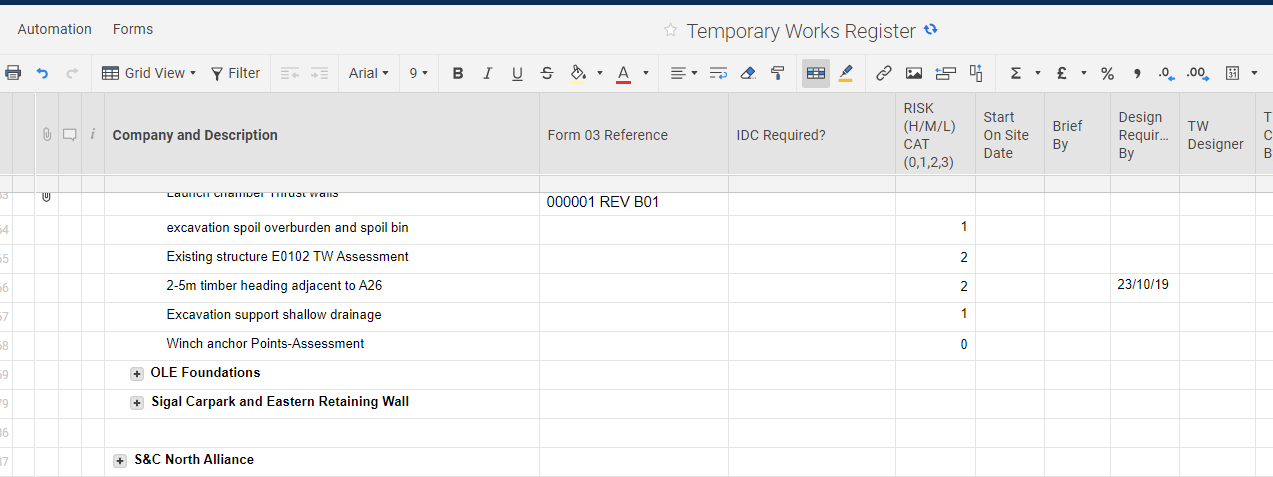or Explore Discussions

# Smartsheet formulas

10/31/19 Edited 12/09/19

Hi,

Can anyone suggest me with a formula to carry out the following function:

- If RISK(H/M/L) CAT (0,1,2,3) column states "2" then Start on site date column needs to insert a date 12 weeks prior to the design required date. If RISK(H/M/L) CAT (0,1,2,3) column states "1", then Start on site date column needs to insert a date 4 weeks prior to the design required date.

This is required to be one formula. Please see attached image of layout of my Smartsheet.Popular Tags:

• Give this a shot...

=IF(ISDATE([Design Required By]@row), [Design Required By]@row - IF([RISK(H/M/L) CAT (0,1,2,3)]@row = 2, 84, 28)

• Sorry that doesn't work. I think I got the question wrong. The formula that I need is if CAT 2 then brief by column needs to insert a date 12 weeks prior. If CAT 1 then 4 weeks prior. Can you please tell me what would the formula me for this case? Also, I didn't understand what @row=2,84,28 meant in your above comment.• The @row replaces the row number and tells the formula to just look at the column specified on whatever row the formula is on. It makes formulas more efficient and avoids the worry of accidentally typing in the wrong row number.

Here's a breakdown of the formula, but judging by your screenshot, it will need adjusted to accommodate more than 2 different selections.

.

=IF(ISDATE([Design Required By]@row), [Design Required By]@row - IF([RISK(H/M/L) CAT (0,1,2,3)]@row = 2, 84, 28))

.

=IF(ISDATE([Design Required By]@row),

This part means says to run the formula if the cell in the row the formula is on in the [Design Required By] column is a date.

.

[Design Required By]@row -

If it is a date, then we want to take that date and subtract a number of days.

.

IF([RISK(H/M/L) CAT (0,1,2,3)]@row = 2,

If the [RISK(H/M/L) CAT (0,1,2,3)] column on the formula row equals 2

.

84,

Then output 84 (days which is 12 weeks to subtract from the original date).

.

28))

Otherwise (if the above mentioned cell does not equal 2) output 28 (days which is 4 weeks to subtract from the original date).

.

.

I do see that you also have a zero in your screenshot though which leads me to believe there is the possibility of the CAT also being a 3.

The formula I have provided should give you a date 12 weeks prior for the CAT being a 2 and 4 weeks for the CAT being a 1.

.

If you need to account for 0 and 3, let me know. Otherwise the formula provided should be working. Can you copy/paste the formula that is giving you an error?

• Thank you so much Paul!

• Happy to help!• Paul, please can you reply to my other two recent posts about Smartsheet formulas? A quick response will be greatly appreciated.

• Can you provide links to the posts in question? I can't promise that I'll be able to help, but I will definitely take a look...

• I have replied to both.